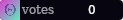Explore# CalcBot

4.67

4.41K

An advanced math calculator with complex numbers, unit conversion, regression analysis, graphing, and more.# Basic calculator usage

The most frequently used command is `c-calculate`, `c-c` for short. Type `c-calculate` followed by an expression to get the answer:

`c-calculate 3*4` -> `12`

`c-calculate (11-4)*2` -> `14`

You can declare variables and use them in later calculations. The special variable `ans` represents the result of the last calculation you made:

`c-calculate x=5` -> `5`

`c-calculate y=x^2` -> `25`

`c-calculate ans` -> `25`

`c-calculate x+y` -> `30`

You can also define custom functions:

`c-calculate quadraticformula(a, b, c) = (-b + sqrt(b^2 - 4 * a * c)) / (2a)`

`c-calculate quadraticformula(1, 5, 6)` -> `-2`

CalcBot supports a wide variety of functions and constants; you can find the full list here.

`c-calculate sin(pi/2)` -> `1`

`c-calculate log(10)` -> `1`

`c-calculate ln(e)` -> `1`

`c-calculate log(8, 2)` -> `3`

`c-calculate sqrt(16)` -> `4`

`c-calculate root(6, 729)` -> `3`

`c-calculate sqrt(-4)` -> `2i`

`c-calculate 6!` -> `720`

`c-calculate phi` -> `1.618033988749895`

# Features

• Powerful scientific calculator (`c-calculate`) with complex number support
• Many computer algebra features, with derivative / integral calculation (`c-calculate derivative`, `c-calculate integral`), root-finding (`c-calculate newtonmethod`), limit estimation (`c-calculate limit`), simplification, etc.
• Linear / polynomial regression analysis with `c-regression` (`c-regression linear` / `c-regression polynomial`)
• Render beautiful graphs with (`c-graph quick` or `c-graph create`)
• Unit conversions with support for custom ratios (`c-unitconvert` / `c-uc`)
• Polynomial and vector modules (`c-polynomial`, `c-vector`)
• Find recently deleted / edited messages (`c-recollect`)
• Run `c-help` for more information and examples for each command

CalcBot is constantly being developed! A full update log is available on my support server. If you'd like to report an issue or suggest a feature, run `c-report` (which are publicly visible on my support server), `c-anonreport` (your username will not be sent with the request) or message me directly at POOLED#9848!

NOTE: If CalcBot does not respond to `c-help` upon invitiation, make sure CalcBot has permissions to send in the server / channel.

## Ratings & Reviews

4.67

9 reviews

Reviews can be left only by registered users. All reviews are moderated by Top.gg moderators. Please make sure to check our guidelines before posting.

5 stars

7

4 stars

1

3 stars

1

2 stars

0

1 star

0Nezu?

almost 3 years ago

Mhm good

5

Details

Prefix

c-

Server Count

4411

Socials

Discord Support Server

Tags

Customizable Behavior

Fun

Utility

CreatorsPOOLED